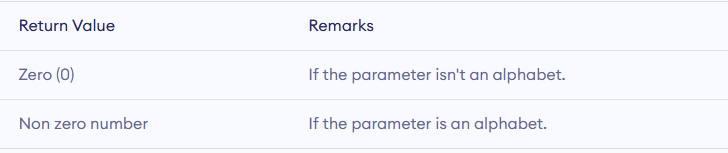## Example program for isupper() function in c

As it's name says isupper() function in c programming checks whether given character is an uppercase alphabet (A-Z) or not.

C isupper() syntax : -

`int isupper(int argument);`
• isupper() function takes a single argument in the form of int.
• Given argument converted into to its ASCII for the check.
•  isupper() define in <ctype.h> header file.

# C isupper() Function Return Valueisupper in c

## Example program for C isupper() function

``````#include <stdio.h>
#include <ctype.h>
int main()
{
char c;

c = 'C';
printf("Return value when uppercase character %c is passed to isupper(): %d", c, isupper(c));

c = '+';
printf("\nReturn value when another character %c is passed to is isupper(): %d", c, isupper(c));

return 0;
}``````

## Example program for isalnum() function in c

The isalnum() function in C use to checks given argument  is an alphanumeric character (alphabet or number) or not.

Syntax : -

`int isalnum(int argument);`

This function is define in ctype.h header file.

## Return Value For isalnum() function in c

it return 1 if given argument in program is an alphanumeric character.

isalnum() function return 0 if given argument in program is not an alphabet or a digit.

# Example program for isalnum() function in c

``````#include <stdio.h>
#include <ctype.h>
int main()
{
char c;
int result;

c = '5';
result = isalnum(c);
printf("When %c is passed, return value is %d\n", c, result);

c = 'Q';
result = isalnum(c);
printf("When %c is passed, return value is %d\n", c, result);

c = 'l';
result = isalnum(c);
printf("When %c is passed, return value is %d\n", c, result);

c = '+';
result = isalnum(c);
printf("When %c is passed, return value is %d\n", c, result);

return 0;
}``````

Output of Program

• When 5 is passed, return value is 1
• When Q is passed, return value is 1
• When l is passed, return value is 1
• When + is passed, return value is 0

## Example Program for isdigit() in c - Function in c

isdigit() function in C programming use to checks whether a character is numeric character (0-9) or not.

# Prototype of isdigit() Function in C

`int isdigit( int arg );`

• isdigit() function in c programming take a single argument which is an integer and return type int.
• Given character is converted to its ASCII value in isdigit() Function
• It is defined in <ctype.h> header file in c.

## Return value for C isdigit()isdigit() Return value in c

# Example Program for isdigit() function in c

``````#include <stdio.h>
#include <ctype.h>

int main()
{
char c;
c='5';
printf("Result when numeric character is passed: %d", isdigit(c));

c='+';
printf("\nResult when non-numeric character is passed: %d", isdigit(c));

return 0;
}``````

OUTPUT : -

```Result when numeric character is passed: 1
Result when non-numeric character is passed: 0```

## Example program for isalpha in c - Function In C

The isalpha() function in  programming is used to check whether a character is an alphabet or not.

If a character is prove that it is an is an alphabet with the help of isalpha() function in c then it return non zero integer otherwise it gives zero.

This function is define in the <ctype.h> header file in c.

## C isalpha() Prototype

`int isalpha(int argument);`

isalpha() function in c takes a single argument in the form of integer and give an integer value.

# Return Value For isalpha() in CReturn Value For isalpha()

## Example Program: C isalpha() function

``````#include <stdio.h>
#include <ctype.h>
int main()
{
char c;
c = 'Q';
printf("\nResult when uppercase alphabet is passed: %d", isalpha(c));

c = 'q';
printf("\nResult when lowercase alphabet is passed: %d", isalpha(c));

c='+';
printf("\nResult when non-alphabetic character is passed: %d", isalpha(c));

return 0;
}``````
``OUTPUT : - ``
``````Result when uppercase alphabet is passed: 1
Result when lowercase alphabet is passed: 2
Result when non-alphabetic character is passed: 0 ``````
`` ``